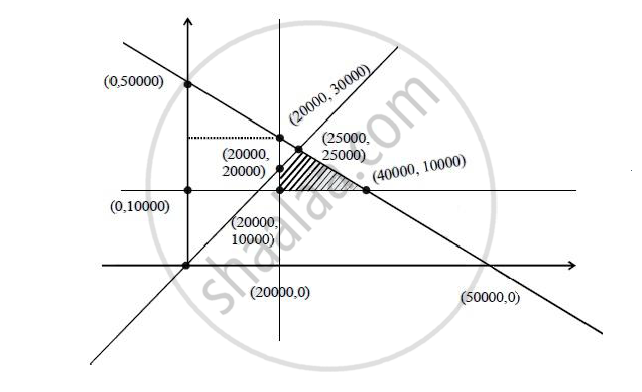# A retired person wants to invest an amount of Rs. 50, 000. His broker recommends investing in two type of bonds ‘A’ and ‘B’ yielding 10% and 9% return respectively on the invested amount. - Mathematics

A retired person wants to invest an amount of Rs. 50, 000. His broker recommends investing in two type of bonds ‘A’ and ‘B’ yielding 10% and 9% return respectively on the invested amount. He decides to invest at least Rs. 20,000 in bond ‘A’ and at least Rs. 10,000 in bond ‘B’. He also wants to invest at least as much in bond ‘A’ as in bond ‘B’. Solve this linear programming problem graphically to maximise his returns.

#### Solution

Maximize Z = 0.1x + 0.09 y

x + y ≤ 50000

x ≥ 20000

y ≥ 10000

y ≤ xz = 0.1 x + 0.09y P1 (20000, 10000) P2 (40000, 10000) P3 (25000, 25000) P4 (20000, 20000) 2900 4900 4750 3800

When A invest 40000 & B invest 10000 his return is maximum.

Concept: Graphical Method of Solving Linear Programming Problems
Is there an error in this question or solution?
2015-2016 (March) All India Set 1 N

Share